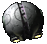## What is the most useless code you can write?

Talk about anything not related to Transcendence.
0xABCDEF
Militia LieutenantPosts: 121
Joined: Thu May 19, 2016 12:58 am
Location: Was destroyed by a Phobos-class dreadnought in the Eridani system
Lately, I have been reading stories of programming horror, where people have to deal with terrible code. Here is one particularly interesting Reddit post about how not to do object-oriented programming. After reading stories like that, I began to wonder about what is the worst code that I could make. Below is one completely useless function that I wrote. It takes the dubious assumption that it usually takes 100 tries to get a random number between 1 and 100 that is equal to 1 and takes it excessively far.

Code: Select all

``````(block
(block Nil
(setq random2a (lambda (min max) (random2b min max min)))
(setq random2b (lambda (min max result) (if (gr (random min max) min) (random2 min max (+ result 1)) result)))
(setq random3a (lambda (min max) (random3b min max min)))
(setq random3b (lambda (min max result) (if (gr (random2a min max) min) (random3b min max (+ result 1))
)
``````
This example creates an integer by repeatedly adding 1

Code: Select all

``````(setq int (lambda (num) (block ((result 0)) (for i 1 num (setq result (+ result (random 1 1)))))))
``````
Here is one that creates a string from another string by individually concatenating each character from the original string to a new one.

Code: Select all

``````(setq string (lambda (str) (block (result) (for i 0 (- (count str) 2) (setq result (cat result (subset str i (+ i 1)))) result))))
``````
And this function destroys the entire API by setting everything, including itself, to Nil

Code: Select all

``````(setq bugCreate (lambda Nil (block (z) (setq z set) (enum (sysGlobals) theFunction (z (cat theFunction) Nil)))))
``````
Here's a function that does nothing

Code: Select all

``````(setq useFul (lambda Nil Nil))
``````
I call the art of writing this horrible code "spaghettification." That's a pun on black holes and spaghetti code. Now, what is the worst code that you can write? Note: All statement calls must affect result in some way or include the result as one of its inputs. I will accept (cat (cat (cat (cat (cat (cat (cat (cat (cat cat))))))))) as an example, but the two extra statements in (block Nil (random 1 1) (random 1 1) (random 1 1)) are simply a waste of space.

digdugPosts: 2615
Joined: Mon Oct 29, 2007 9:23 pm
Location: Decoding hieroglyphics on Tan-Ru-Dorem
I went through my debug mod, and found these gems:

Code: Select all

``````(setq blah (lambda Nil
(block Nil

)))``````
as well as this awesome ship (I swear I used it for something, but I don't remember what it was)

Code: Select all

``````<ShipClass UNID="&evtest;"
class=				"(test)"
virtual=			"true"

>

<Events>
<IMTESTEVENT>
(dbglog "IT WORKS!!!!!!!!!!!!!!")
</IMTESTEVENT>

</Events>
</ShipClass>``````

AssumedPseudonym
Fleet OfficerPosts: 1099
Joined: Thu Aug 29, 2013 5:18 am
Location: On the other side of the screen.
Somewhere between useless and trololololol:

Code: Select all

``````(setq aeOopsIBrokeIt
(lambda nil
(plyDestroyed gPlayer
(cat "debugged " (if (strFind (plyGetGenome gPlayer) 'female) "her" "him") "self to death")
)
)
)``````Mod prefixes: 0xA010 (registered) and 0xDCC8 (miscellaneous)